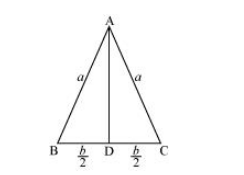# The two equal sides of an isosceles triangle with fixed base b are decreasing`
Question:

The two equal sides of an isosceles triangle with fixed base b are decreasing at the rate of 3 cm per second. How fast is the area decreasing when the two equal sides are equal to the base?

Solution:

Let ΔABC be isosceles where BC is the base of fixed length b.

Let the length of the two equal sides of ΔABC be a.

Draw $A D \perp B C$.Now, in ΔADC, by applying the Pythagoras theorem, we have:

$\mathrm{AD}=\sqrt{a^{2}-\frac{b^{2}}{4}}$

$\therefore$ Area of triangle $(A)=\frac{1}{2} b \sqrt{a^{2}-\frac{b^{2}}{4}}$

The rate of change of the area with respect to time (t) is given by,

$\frac{d A}{d t}=\frac{1}{2} b \cdot \frac{2 a}{2 \sqrt{a^{2}-\frac{b^{2}}{4}}} \frac{d a}{d t}=\frac{a b}{\sqrt{4 a^{2}-b^{2}}} \frac{d a}{d t}$

It is given that the two equal sides of the triangle are decreasing at the rate of 3 cm per second.

$\therefore \frac{d a}{d t}=-3 \mathrm{~cm} / \mathrm{s}$

$\therefore \frac{d A}{d t}=\frac{-3 a b}{\sqrt{4 a^{2}-b^{2}}}$

Then, when b, we have:

$\frac{d A}{d t}=\frac{-3 b^{2}}{\sqrt{4 b^{2}-b^{2}}}=\frac{-3 b^{2}}{\sqrt{3 b^{2}}}=-\sqrt{3} b$

$\frac{d A}{d t}=\frac{-3 b^{2}}{\sqrt{4 b^{2}-b^{2}}}=\frac{-3 b^{2}}{\sqrt{3 b^{2}}}=-\sqrt{3} b$

Hence, if the two equal sides are equal to the base, then the area of the triangle is decreasing at the rate of $\sqrt{3} b \mathrm{~cm}^{2} / \mathrm{s}$.